• 2020-11-26 08:32:15

#coding:UTF-8

"""

Python

implementation

of

Haversine

formula

(C)

<2009>

Bartek

Górny

This

program

is

free

software:

you

can

redistribute

it

and/or

modify

it

under

the

terms

of

the

GNU

General

Public

as

published

by

the

Free

Software

Foundation,

either

version

3

of

the

or

(at

your

option)

any

later

version.

This

program

is

distributed

in

the

hope

that

it

will

be

useful,

but

WITHOUT

ANY

WARRANTY;

without

even

the

implied

warranty

of

MERCHANTABILITY

or

FITNESS

FOR

A

PARTICULAR

PURPOSE.

See

the

GNU

General

Public

for

more

details.

You

should

have

a

copy

of

the

GNU

General

Public

along

with

this

program.

If

not,

see

.

"""

import

math

def

recalculate_coordinate(val,

_as=None):

"""

Accepts

a

coordinate

as

a

tuple

(degree,

minutes,

seconds)

You

can

give

only

one

of

them

(e.g.

only

minutes

as

a

floating

point

number)

and

it

will

be

duly

recalculated

into

degrees,

minutes

and

seconds.

Return

value

can

be

specified

as

'deg',

'min'

or

'sec';

default

return

value

is

a

proper

coordinate

tuple.

"""

deg,

min,

sec

=

val

#

pass

outstanding

values

from

right

to

left

min

=

(min

or

0)

+

int(sec)

/

60

sec

=

sec

%

60

deg

=

(deg

or

0)

+

int(min)

/

60

min

=

min

%

60

#

pass

decimal

part

from

left

to

right

dfrac,

dint

=

math.modf(deg)

min

=

min

+

dfrac

*

60

deg

=

dint

mfrac,

mint

=

math.modf(min)

sec

=

sec

+

mfrac

*

60

min

=

mint

if

_as:

sec

=

sec

+

min

*

60

+

deg

*

3600

更多相关内容
• 计算两点(x1,y1),(x2,y2)之间的距离，输出并保留一位小数 两点之间距离的数学公式： txt=input("请输入四个数字，用空格隔开:") ls=txt.split(" ") x1=eval(ls) y1=eval(ls) x2=eval(ls) y2=eval(ls) r...

从键盘输入4个数字，采用空格分隔，对应变量为x1,y1,x2,y2。计算两点(x1,y1),(x2,y2)之间的距离，输出并保留一位小数

两点之间距离的数学公式：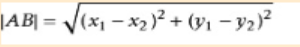txt=input("请输入四个数字，用空格隔开:")
ls=txt.split(" ")
x1=eval(ls)
y1=eval(ls)
x2=eval(ls)
y2=eval(ls)
r=pow(pow(x1-x2,2)+pow(y1-y2,2),0.5)
print("{:.1f}".format(r))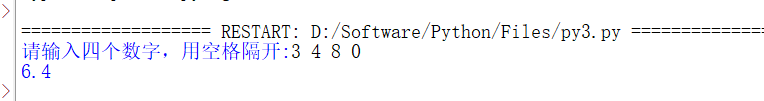展开全文开发语言 后端
• 已知地球上任意两点（lng1，lat1），（lng2， lat2）的经纬度坐标，求两点间的距离可以利用 haversine 公式： 首先先将经纬度坐标的角度化成弧度（rlng1，rlat1），（rlng2，rlat2) 利用如下公式： 其中 a ...# 一、基本原理

处理地理数据时，经常需要用到两个地理位置间的距离。比如 A 点经纬度（30.553949，114.357399），B点经纬度（129.1344，25.5465），求 AB 两点之间的距离。

已知地球上任意两点（lng1，lat1），（lng2， lat2）的经纬度坐标，求两点间的距离可以利用 haversine 公式：

• 首先先将经纬度坐标的角度化成弧度（rlng1，rlat1），（rlng2，rlat2)

• 利用如下公式：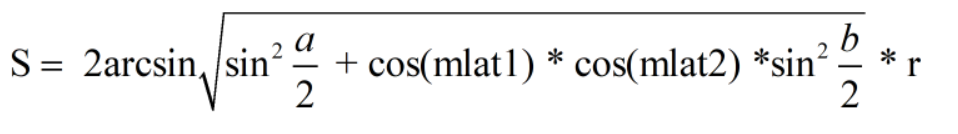• 其中 a 表示两点维度的差值，即 a = rlat1 - rlat2， b表示两点经度的差值，即 b = rlng1 - rlng2；其中 r 表示地球的半径

网址：

展开全文haversine geopy
• #定义的函数 class Point: def __init__(self,x=0,y=0): self.x=x self.y=y def getx(self): return self.x def gety(self): return self.y #定义直线函数 class Getlen: def __init__(self,p1,p2): self...
• 算了，找了半天，就用第一种方法吧 dis = math.hypot(10,10) 计算和0,0之间的距离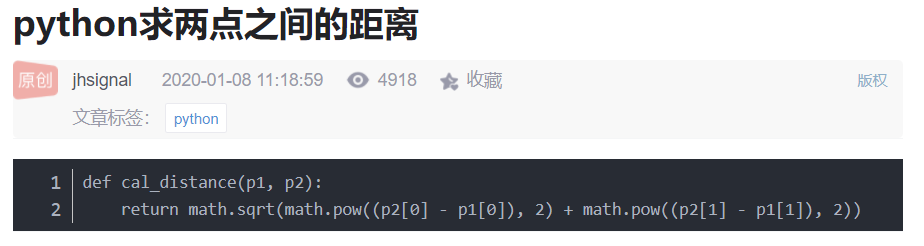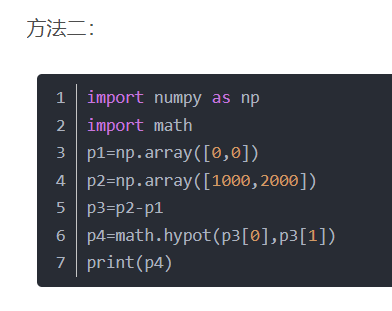算了，找了半天，就用第一种方法吧

dis = math.hypot(10,10)

计算该点和0,0之间的距离

展开全文• result2 = list(nearest_points(a, b)....生成的result2对应的为b 上距离 a 最近的 result2 = list(nearest_points(a, b).coords) 生成的result2对应的为a 上距离 b 最近的开发语言 后端
• 我有张单子。每个列表有三行。每个列表的坐标系从上到下为(x，y，z)。我试着用数组，但没用。这是我的密码。在import numpy as npp1 = np.array([list(marker_11_x['11:-.X']), list(marker_11_y['11:-.Y']),list...
• 计算Python Numpy向量之间的欧氏距离，已知vec1和vec2是个...# 补充知识：Python计算两个数据之间的欧式距离，一个到数据集中其他距离之和 # 如下所示： # 计算个数据之间的欧式距离 import npytorch
• 平常为了得出地理位置上两点的实际距离（譬如北京与杭州之间的实际距离），除了利用经纬度计算两点空间距离，还需要考虑地形因素。由于之间考虑地形造成误差较大，因此采用微分的办法来解决，简单来说就是将两点...算法
• 类似的需求应该比较多，这里的核心是关于个坐标之间距离计算，之前没用过这个东西，刚好今天有个这样的需求，记录下。 import requests import psycopg2 import pandas as pd from math import radians, ...
• 闵可夫斯基距离(Minkowski Distance)2.欧氏距离(Euclidean Distance)3.曼哈顿距离(Manhattan Distance)4.切比雪夫距离(Chebyshev Distance)5.夹角余弦(Cosine)6.汉明距离(Hamming distance)7.杰卡德相似系数(Jaccard...
• #coding:UTF-8"""Python implementation of Haversine formulaCopyright (C) <2009> Bartek GórnyThis program is free software: you can redistribute it and/or modifyit under the terms of the GNU ...
• 利用python计算两空间直线的公垂线距离并计算和条直线之间的交点
• 如图为一个10*10的网格图，黑点处为障碍物，给定任意两点——start和end，求最短路径，走一格距离为1。 Code # 算法思想：通过BFS找end，找到就停 # 部分变量含义： # value->网格图列表 # hinders->障碍点...算法 广度优先
• 这是来自知乎上的问题。问我的时候，恰好我在写一个和向量计算相关的文章，于是灵光乍现，顺手写了这样一个答案。该算法未经严格验证，请谨慎参考。numpy
• 通过调用geographiclib包的函数，一行代码即可返回个经纬度之间的距离和方位角/夹角，非常快速高效，省去补习立体空间几何之烦恼。本文也详细讲解了求距离和角度的数学原理，有图有公式，适合深入研究者参考。
• 在数学中，欧几里得距离或欧几里得度量是欧几里得空间两点间“普通”（即直线）距离。使用这个距离，欧氏空间成为度量空间。 （图片摘自百度百科） 欧式距离是两个图形最近点的距离（图中红点的距离，不会考虑...
• 任意维度两点距离计算任意维坐标中两点之间距离:import math def distance(p0,p1,digits=2): a=map(lambda x: (x-x...
• 中学数学告诉我们，计算点到线段的距离种。 我们假设是P，线段是AB。P和AB都由三维的笛卡尔坐标表示。现计算P到AB的距离。 第一种是，过P向线段AB上画垂线，判断垂足有没有落在线段上。如果落在线段上，ok...
• 1、当个集合元素个数相同，则直接调包 from numpy import * import scipy.spatial.distance as dist # 导入scipy距离公式 matV = mat([[1,1,0,1,0,1,0,0,1],[0,1,1,0,0,0,1,1,1]]) print ("dist.jaccard:", dist...
• 在分析数据时，有时要计算流形上两点之间的测地距离。本着有现成轮子绝对不自己写的观点，发现可以通过以下方式计算流形上任意两点之间的测地距离。 ISOMAP是一种保持测地距离不变的高维空间中低维流形的降维方法。...
• 所列的距离公式列表和代码如下：闵可夫斯基距离(Minkowski Distance)欧氏距离(Euclidean Distance)曼哈顿距离(Manhattan Distance)切比雪夫距离(Chebyshev Distance)夹角余弦(Cosine)汉明距离(Hamming distance)...
• #计算公式 def getlen(self): return self.len #得到距离·1 p1 = Point(3,4,5) #设置p1坐标 p2 = Point(7,9,11) #设置p2坐标 l = Getlen(p1,p2) #定义对象 print(l.getlen()) #输出 import math class Point: def _...
• 欧式距离能够体现个体数值特征的绝对差异，所以更多的用于需要从维度的数值代销中体现差异 余弦距离更多的是从方向上区分差异，而对绝对的数值不敏感，更多的用于使用用户对内容评分类区分兴趣的相似度和差异 同时...
• 题目如下： 程序如下： import math def distance(a,b,c): d=pow(a**2+b**2+c**2,1/2) return d x,y,z=input().split(",") d=distance(float(x),float(y),float(z)) print("{:.2f}".format(d)) ...3008算法
• python实现到平面的距离 目录 python实现到平面的距离 1.三点定面 2.到面的距离 3.python实现到面的距离 关于线面之间关系可以参考:https://blog.csdn.net/qq_23869697/article/details/82688277 1...
• 近期在用 OpenCV 时需要... 到直线距离由于项目中得到的坐标最容易，因此采用向量法进行所有的数学计算最清晰明了。到直线距离就采用向量法推导。 推导过程： 代码实现：array_longi = np.array([x2-x1, y...
• 这个时候将二维坐标转化为复数的话那么就可以使用python中的abs绝对值函数计算两个点之间的距离或者是计算复数的模，当我们将两个复数对应的坐标相减然后对其使用abs绝对值函数那么得到的就是两点之间的距离（对复数......